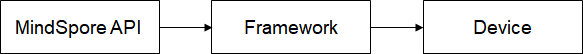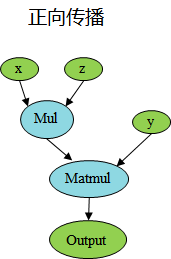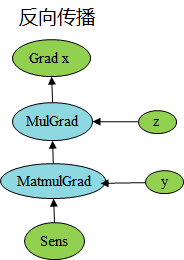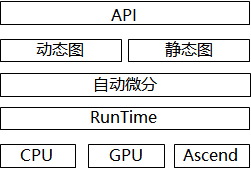# 动静态图结合¶

## MindSpore静态图¶

### Graph模式执行原理¶

Graph模式的代码用例如下所示：

:

import numpy as np
import mindspore.nn as nn
import mindspore.ops as ops
import mindspore as ms

ms.set_context(mode=ms.GRAPH_MODE, device_target="CPU")

class Net(nn.Cell):
def __init__(self):
super(Net, self).__init__()
self.mul = ops.Mul()

def construct(self, x, y):
return self.mul(x, y)

x = ms.Tensor(np.array([1.0, 2.0, 3.0]).astype(np.float32))
y = ms.Tensor(np.array([4.0, 5.0, 6.0]).astype(np.float32))

net = Net()
print(net(x, y))

[ 4. 10. 18.]


## MindSpore动态图¶

### PyNative模式执行原理¶:

import numpy as np
import mindspore.nn as nn
import mindspore as ms
import mindspore.ops as ops

ms.set_context(mode=ms.PYNATIVE_MODE, device_target="CPU")
x = ms.Tensor(np.ones([1, 3, 3, 4]).astype(np.float32))
y = ms.Tensor(np.ones([1, 3, 3, 4]).astype(np.float32))
print(output.asnumpy())

[[[[2. 2. 2. 2.]
[2. 2. 2. 2.]
[2. 2. 2. 2.]]

[[2. 2. 2. 2.]
[2. 2. 2. 2.]
[2. 2. 2. 2.]]

[[2. 2. 2. 2.]
[2. 2. 2. 2.]
[2. 2. 2. 2.]]]]


### PyNative模式自动微分原理¶

:

import numpy as np
import mindspore.nn as nn
import mindspore.ops as ops
import mindspore as ms

ms.set_context(mode=ms.PYNATIVE_MODE, device_target="CPU")

class Net(nn.Cell):
def __init__(self):
super(Net, self).__init__()
self.matmul = ops.MatMul()
self.z = ms.Parameter(ms.Tensor(np.array([2.0], np.float32)), name='z')

def construct(self, x, y):
x = x * self.z
out = self.matmul(x, y)
return out

def __init__(self, net):
self.net = net

def construct(self, x, y):

x = ms.Tensor([[0.8, 0.6, 0.2], [1.8, 1.3, 1.1]], dtype=ms.float32)
y = ms.Tensor([[0.11, 3.3, 1.1], [1.1, 0.2, 1.4], [1.1, 2.2, 0.3]], dtype=ms.float32)
print(output)

[[9.02      5.4       7.2000003]
[9.02      5.4       7.2000003]]:

from mindspore.ops._grad.grad_base import bprop_getters

@bprop_getters.register(ops.Mul)
def get_bprop_mul(self):
"""Grad definition for Mul operation."""
mul_func = P.Mul()

def bprop(x, y, out, dout):
bc_dx = mul_func(y, dout)
bc_dy = mul_func(x, dout)

return bprop


## 动静统一¶

### 概述¶### 动态图和静态图互相转换¶

:

ms.set_context(mode=ms.PYNATIVE_MODE)


### 动静结合¶

MindSpore支持在动态图下使用静态编译的方式来进行混合执行，通过使用jit修饰需要用静态图来执行的函数对象，即可实现动态图和静态图的混合执行，更多jit的使用可参考jit文档

:

import numpy as np
import mindspore as ms
import mindspore.nn as nn

def __init__(self):
self.param = ms.Parameter(ms.Tensor(0.5, ms.float32))

@ms.jit
def construct(self, x):
x = x + x
x = x * self.param
x = x * x
return x

class CellCallSingleCell(nn.Cell):
def __init__(self):
super(CellCallSingleCell, self).__init__()
self.bn = nn.BatchNorm2d(2, momentum=0.99, eps=0.00001, gamma_init="ones")
self.relu = nn.ReLU()

def construct(self, x):
x = self.conv(x)
x = self.bn(x)
x = self.relu(x)
return x

ms.set_context(mode=ms.PYNATIVE_MODE, device_target="CPU")
inputs = ms.Tensor(np.ones([1, 1, 2, 2]).astype(np.float32))
net = CellCallSingleCell()
out = net(inputs)
print(out)

[[[[15.99984]]

[[15.99984]]]]


### JIT Fallback¶

JIT Fallback是为了实现动静统一提出的功能特性。通过JIT Fallback等特性，静态图可以支持尽量多的动态图语法，使得静态图提供接近动态图的语法使用体验。

JIT Fallback是从静态图的角度出发考虑静态图和动态图的统一。在MindSpore静态图模式下，用户编写程序时需要遵循MindSpore静态图语法支持，语法使用存在约束限制。而在动态图模式下，Python脚本代码会根据Python语法进行执行，用户可以使用任意Python语法。可以看出，静态图和动态图的语法约束限制是不同的。JIT Fallback特性可以使得静态图支持尽量多的动态图语法，用户能够灵活地进行静态图和动态图的切换。

:

import numpy as np
import mindspore.nn as nn
import mindspore as ms

ms.set_context(mode=ms.GRAPH_MODE, device_target="CPU")

class Net(nn.Cell):
def construct(self):
x = np.array([1, 2, 3])
y = ms.Tensor(x)
return y

net = Net()
print(net())

[1 2 3]